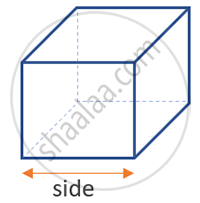Share

# Surface Area and Volume of Cube, Cuboid and Cylinder - Concept of Cube

#### notes

A cube is a 3d representation of a square and has all equal sides. The length, breadth, and height in a cube are the same and are termed as sides (s). If s is each edge of the cube then,
the surface area of a cube=2 [(s × s) + (s × s) + (s × s)] = 2(3 s^2 ) = 6 s^2.Lateral Surface Area of Cube :
The area of 4 constituting squares gives us the lateral surface area of the cube.
So the lateral surface area of the cube shall be s^2+s^2+s^2+s^2 = 4 s^2.
The lateral surface area of a cube = 4 s^2

S